Courses

# Test: Electromagnetic Waves - From Past 28 Years Questions

## 27 Questions MCQ Test Physics Class 12 | Test: Electromagnetic Waves - From Past 28 Years Questions

Description
This mock test of Test: Electromagnetic Waves - From Past 28 Years Questions for Class 12 helps you for every Class 12 entrance exam. This contains 27 Multiple Choice Questions for Class 12 Test: Electromagnetic Waves - From Past 28 Years Questions (mcq) to study with solutions a complete question bank. The solved questions answers in this Test: Electromagnetic Waves - From Past 28 Years Questions quiz give you a good mix of easy questions and tough questions. Class 12 students definitely take this Test: Electromagnetic Waves - From Past 28 Years Questions exercise for a better result in the exam. You can find other Test: Electromagnetic Waves - From Past 28 Years Questions extra questions, long questions & short questions for Class 12 on EduRev as well by searching above.
QUESTION: 1

### Which of the following, is the longest wave ?       

Solution:

Rays  -    Wavelength
[Range in m]
X-rays    -  1 × 10–11 to 3 × 10–8
γ-rays     -  6 × 10–14 to 1 × 10–11
Microwaves    -  10–3 to 0.3

QUESTION: 2

### Pick out the longest wavelength from the following types of radiation. 

Solution:

Wavelength of red light is longest

QUESTION: 3

### The frequency of electromagnetic wave, whichis best suited to observe a particle of radius3 × 10–4 cm is of the order of             

Solution:

The Wave length of radiation used should be less than the size of the particle =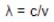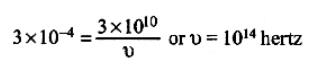QUESTION: 4

The structure of solids is investigated by using        

Solution:

X-rays are used for the investigation of
structure of solids

QUESTION: 5

A signal emitted by an antenna from a certainpoint can be received at another point of thesurface in the form of                

Solution:
QUESTION: 6

The oscillating electric and magnetic field vectorsof electromagnetic wave are oriented along   

Solution:

The direction of oscillations of E and B fields are perpendicular to each other as well as to the direction of propagation. So, electromagnetic waves are transverse in nature. The electric and magnetic fields oscillate in same phase.

QUESTION: 7

Which one of the following electromagneticradiations has the smallest wavelength? 

Solution:

Rays  -    Wavelength
[Range in m]
X-rays    -  1 × 10–11 to 3 × 10–8
γ-rays     -  6 × 10–14 to 1 × 10–11
Microwaves    -  10–3 to 0.3

QUESTION: 8

If ∈0 and μ0 are the electric permittivity and  magnetic permeability in vacuum, ∈ and μ are corresponding quantities in medium, then refractive index of the medium is 

Solution:

We know that velocity of  lectromagnetic
wave in vacuum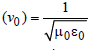velocity of electromagnetic wave in
medium is (v) =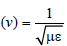Therefore refractive index of the medium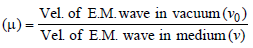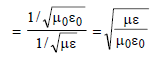QUESTION: 9

​In a circuit, inductance L and capacitance C are connected as shown in the figure. A1 and A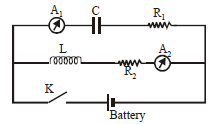are ammeters. When key K is pressed to complete the circuit, then just after closing key (K), the readings of A1 and A2 will be          

Solution:

Initially there is no D.C. current in inductive circuit and maximum D.C. current is in capacitive current. Hence, the current is zero in A2 and maximum in A1.

QUESTION: 10

The electromagnetic radiations are caused by       

Solution:

A stationary charge produces electric field only; an uniformly moving charge produces localised electromagnetic field; an accelerated charge produces electromagnetic radiations.

QUESTION: 11

The frequencies of X-rays, γ-rays and ultravioletrays are respectively a, b, and c. Then      

Solution:

γ rays has lowest wavelength and highest frequency among them while ultraviolet ray has highest wavelength and lowest frequency.
Order of frequency : b > a > c

QUESTION: 12

Which of the following is positively charged?      

Solution:

α rays contain Helium nuclei which contains 2 unit of positive charge.

QUESTION: 13

if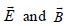represent electric and magnetic field vectors of the electromagnetic waves, then the direction of propagation of the waves will be along

Solution:

Direction of propagation of electro-magnetic waves is perpendicular to Electric field and Magnetic field. Hence, direction is given by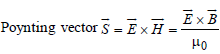QUESTION: 14

Which of the following electromagneticradiations has the least wavelength? 

Solution:

Gamma ray has highest frequency and lowest wavelength.

QUESTION: 15

Green-house effect is the heating up of earth's atmosphere due to         

Solution:

Infrared rays is the cause of Green house effect. The glass transmits visible light and short infrared rays which are absorbed by plants. Then it emits long infrared rays, which are reflected back by glass.

QUESTION: 16

Which one of the following rays is notelectromagnetic wave ? 

Solution:

β ray is not electromagnetic ray

QUESTION: 17

We consider the radiation emitted by the humanbody. Which of the following statements is true?      

Solution:

Depends on the magnitude of frequency

QUESTION: 18

If λv, λx and λm represent the wavelengths of visible light, X–rays and microwaves respectively, then

Solution:

We know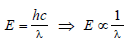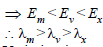QUESTION: 19

The electric and magnetic field of an electromagneticwave are       

Solution:

Variation in magnetic field causes electric field and vice-versa.
In electromagnetic waves,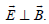.
Both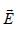and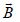are in the same phase.
In electromagnetic waves
E = E0 sin(ωt - kx)
B = B0 sin(ωt - kx)
The electromagnetic waves travel in the
direction of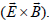QUESTION: 20

The velocity of electromagnetic radiation in a medium of permittivity ∈0 and permeability μ0 is given by 

Solution:

in a medium of permittivity  ∈and
permeability μis equal to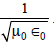QUESTION: 21

The electric field part of an electromagnetic wave in a medium is represented by Ex=0;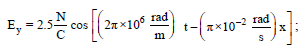Ez = 0. The wave is :               

Solution:

Comparing with the equation of wave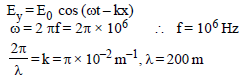QUESTION: 22

Which of the following statement is false for theproperties of electromagnetic waves? 

Solution:

Electromagnetic waves are the combination of mutually perpendicular electric and magnetic fields. So, option (c) is false.

QUESTION: 23

The electric and the magnetic field associated with an E.M. wave, propagating along the +z-axis, can be represented by 

Solution:

The electromagnetic wave is propagating along the +z axis.
Since the electric and magnetic fields are perpendicular to each other and also perpendicular to the direction of propagation of wave.
Also.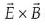gives the direction of wave propagation.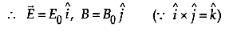QUESTION: 24

The decreasing order of wavelength of infrared,microwave, ultraviolet and gamma rays is 

Solution:

The decreasing order of the wavelengths is as given below :
microwave, infrared, ultraviolet, gamma
rays.

QUESTION: 25

The electric field associated with an e.m. wave in vacuum is given by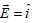cos (kz – 6 ×108t), where E, z and t are in volt/m, meter and seconds respectively. The value of wave vector k is : 

Solution:

On comparing the given equation to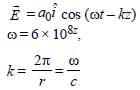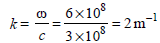QUESTION: 26

The ratio of amplitude of magnetic field to theamplitude of electric field for an electromagneticwave propagating in vacuum is equal to :   [2012M]

Solution:

The average energy stored in the electric field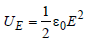The average energy stored in the magnetic field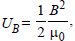According to conservation of energy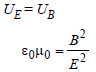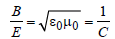QUESTION: 27

An electromagnetic wave of frequency v = 3.0MHz passes from vacuum into a dielectricmedium with relative permittivity ε = 4.0. Then[NEET Kar. 2013]

Solution:

Given: frequency f = 2MHz, relative
permittivity ∈r= 4

From formula,

velocity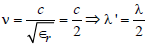[Since frequency remains unchanged]